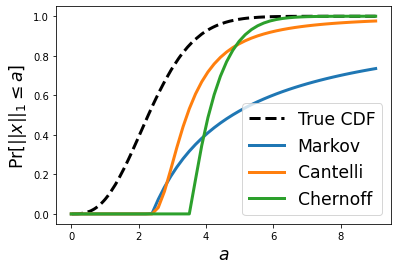## Posts

Showing posts from 2021

### Tail Bounds on the Sum of Half Normal Random VariablesLet $x \sim \mathcal{N}(0, \sigma^2)^n$ be a vector of $n$ i.i.d normal random variables.  We wish to provide a high probability tail bound on the $L_1$ norm of $x$, that is: $$Pr[|| x ||_1 \geq a] \leq f(a)$$ Note that the term on the left is called a "tail probability" because it captures the probability mass in the tail of the distribution (i.e., values exceeding a).  This type of problem arises when we wish to claim that the probability is small for a given random variable to be large.  The tail probability above is closely related to the CDF of the random variable $|| x ||_1$: it is exactly $1 - Pr[|| x ||_1 \leq a]$.  Unfortunately, the CDF of this random variable does not appear to have a clean closed form expression, and for that reason, we wish to find a reasonably clean and tight bound on it instead.  In this blog post, we will explore, utilize, and compare the Markov inequality , the one-sided Chebyshev inequality , and the Chernoff bound to bound the de

### Open Problem: Efficiently Evaluating Subset Sums

Suppose we are given a vector of $n$ items $\begin{bmatrix} x_1 & x_2 & \dots x_n \end{bmatrix}$ and we would like to perform a number of subset sums on these items.  Specifically, we would like to compute $\sum_{i \in S} x_i$ for some collection of subsets $S \subseteq \{ 1, \dots, n \}$.   We would like to evaluate all of the summations using the minimum number of arithmetic operations (additions and subtractions). In this setting, addition and subtraction of two elements is an expensive operation (e.g., think of them as being 2D numpy arrays).  Therefore, we are willing to spend computational time and resources to find an efficient means to evaluate the subset sums.  Moreover, we may need to compute these subset sums many times, e.g., if they are computed as part of an inner loop to an iterative algorithm.  Therefore, the time spent finding the right way to evaluate the subsets sums will ultimately pay for itself. A few examples with solutions to make this problem more co

### Removing redundant constraints from a linear system of equations

Suppose you are solving an optimization problem with linear equality constraints of the form  $Ax = b$ Several off-the-shelf optimizers, including those available in CVXOPT and scipy will complain if A is not full rank.  That is, if A contains redundant constraints then optimization will likely fail.  So how can we easily deal with this issue?  The code below is something you can plug into your program for a quick fix to the issue.  Simply call A, b = reduce_row_echelon(A, b) to get an equivalent set of constraints with redundancies removed.  # Consumes a *consistent* rectangular system of equations # Produces a new compacted system of equations with linearly dependent rows removed # Note: this algorithm computes the row echelon form of the augmented matrix # This algorithm should be numerically robust def reduce_row_echelon(B, y): A = np.concatenate([B, y[:,np.newaxis]], axis=1) m,n = A.shape c = 0 for r in range(m): # find first non-zero column wh

### log-dot-exp: generalizing the log-sum-exp trick

In scientific computing applications we often have to work with very small positive numbers.  With finite precision representation for numbers (like 32 or 64 bit floats), this can be a problem when precision matters even at the smallest scales.  In these cases, it is useful to represent numbers in log space and perform computations directly in that space.  Working in log space can make a numerically unstable algorithm stable.  Suppose for example that we want to know the sum of $n$ positive real numbers: $Z = \phi_1 + \dots + \phi_n$.  In log space, we would instead work with $\theta_i = \log{(\phi_i)}$.  To perform this summation in log space, we need to evaluate the following expression: $$\log Z = \log \sum_{i} \exp{(\theta_i)}$$ When done naively, this computation can be numerically unstable, e.g., if $\theta_i$ is large then $\exp{(\theta_i)}$ may cause an overflow error.  The Log-Sum-Exp  trick provides a way to do this computation in a numerically stable manner.  In particular